Next: Fast Fourier Transform (FFT) Up: SR Spectroscopy Previous: Time Differential SR (TD-SR)   Contents

# Transverse-Field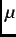SR (TF-SR)

In a transverse-field muon spin rotation experiment (Fig. 2.3), a magnetic field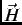is applied perpendicular to the initial muon-spin polarization direction. A muon stopping in the sample Larmor precesses about the local magnetic field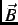(which in general is different than the external field) at an angular frequency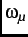=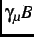, where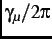=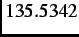MHzT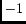is the muon gyromagnetic ratio.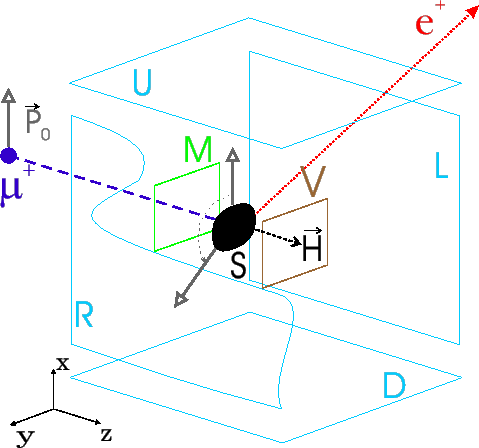In a superconductor the muons stop at well defined sites in the crystal lattice. However, in the vortex state of a type-II superconductor, the muons stop randomly on the length scale of the vortex lattice (which is typically two to three orders of magnitude larger than that of the crystal lattice). Consequently,SR is an effective local probe of the spatial variation of internal magnetic fields due to the periodic arrangement of vortices. For the case where the external field is directed along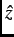, the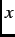component of the muon-spin polarization function is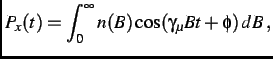(3.6)

where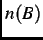is the probability that a muon sees a local magnetic field between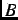and+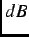, and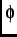is the initial phase. There are several sources of the local magnetic field sensed by the muons. First there is the magnetic field inhomogeneity associated with the vortex lattice. For a type-II superconductor, the spatial profile of the magnetic field in the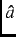-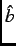plane due to an applied field along the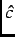axis (direction) is reasonably described by the phenomenological model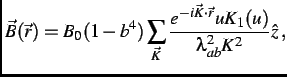(3.7)

where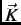is a reciprocal lattice vector,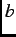=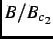is the reduced field,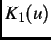is a modified Bessel function and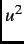=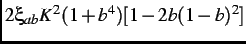. Equation (2.7), which is derived from Ginzburg-Landau theory, assumes that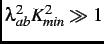, where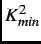is the magnitude of the smallest non-zero reciprocal-lattice vector in the summation. This condition is satisfied for an extreme type-II superconductor like the high-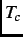cuprates, where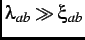. This self-consistent analytic function, agrees extremely well with the exact numerical solutions of the Ginzburg-Landau equations at low reduced fields. Random vortex pinning and thermal fluctuations modify the field distribution associated with Eq. (2.7), which assumes a perfect periodic arrangement of vortices.

The muon is also sensitive to both nuclear and electronic dipole moments. The nuclear moments are randomly oriented at temperatures reachable in aSR experiment (i.e.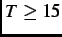mK), whereas electronic magnetic moments may order. In general, the magnetic moments that are static on theSR time scale broaden the measured internal magnetic field distribution. To account for the effects of this additional source of field inhomomgeneity on the muon-spin polarization function, Eq. (2.6) can be multiplied by a depolarization function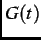such that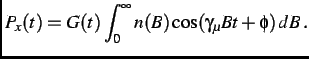(3.8)

The precise functional form ofdepends on the nature of the additional sources of magnetic field at the muon site. For example, a Gaussian function=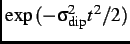with a depolarization rate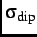, characterizes the damping of the muon-spin precession signal due to dense static dipole moments .Next: Fast Fourier Transform (FFT) Up: SR Spectroscopy Previous: Time Differential SR (TD-SR)   Contents
Jess H. Brewer 2003-07-01